Next: Exercises Up: Hamiltonian Dynamics Previous: Constrained Lagrangian Dynamics

Hamilton's Equations

Consider a dynamical system with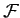degrees of freedom which is described by the generalized coordinates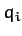, for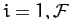. Suppose that neither the kinetic energy,, nor the potential energy,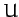, depend explicitly on the time,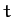. Now, in conventional dynamical systems, the potential energy is generally independent of the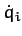, whereas the kinetic energy takes the form of a homogeneous quadratic function of the. In other words,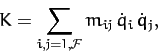(744)

where the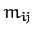depend on the, but not on the. It is easily demonstrated from the above equation that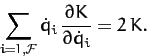(745)

Recall, from Section 9.8, that generalized momentum conjugate to theth generalized coordinate is defined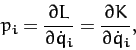(746)

where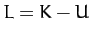is the Lagrangian of the system, and we have made use of the fact thatis independent of the. Consider the function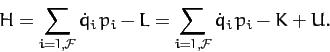(747)

If all of the conditions discussed above are satisfied then Equations (745) and (746) yield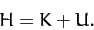(748)

In other words, the function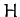is equal to the total energy of the system.

Consider the variation of the function. We have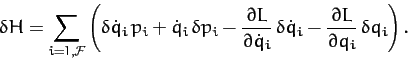(749)

The first and third terms in the bracket cancel, because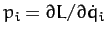. Furthermore, since Lagrange's equation can be written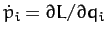(see Section 9.8), we obtain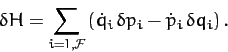(750)

Suppose, now, that we can express the total energy of the system,, solely as a function of theand the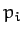, with no explicit dependence on the. In other words, suppose that we can write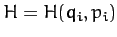. When the energy is written in this fashion it is generally termed the Hamiltonian of the system. The variation of the Hamiltonian function takes the form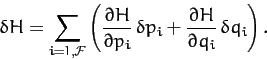(751)

A comparison of the previous two equations yields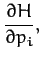(752)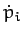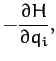(753)

for. These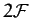first-order differential equations are known as Hamilton's equations. Hamilton's equations are often a useful alternative to Lagrange's equations, which take the form ofsecond-order differential equations.

Consider a one-dimensional harmonic oscillator. The kinetic and potential energies of the system are written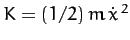and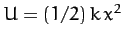, whereis the displacement,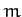the mass, and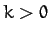. The generalized momentum conjugate tois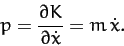(754)

Hence, we can write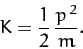(755)

So, the Hamiltonian of the system takes the form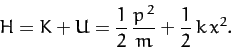(756)

Thus, Hamilton's equations, (752) and (753), yield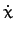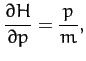(757)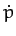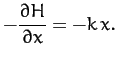(758)

Of course, the first equation is just a restatement of Equation (754), whereas the second is Newton's second law of motion for the system.

Consider a particle of massmoving in the central potential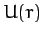. In this case,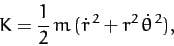(759)

where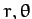are polar coordinates. The generalized momenta conjugate to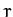andare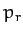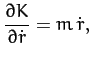(760)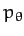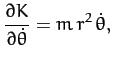(761)

respectively. Hence, we can write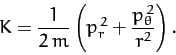(762)

Thus, the Hamiltonian of the system takes the form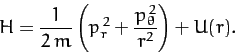(763)

In this case, Hamilton's equations yield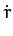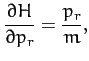(764)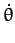(765)

which are just restatements of Equations (760) and (761), respectively, as well as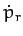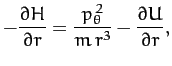(766)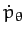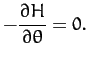(767)

The last equation implies that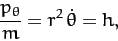(768)

where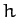is a constant. This can be combined with Equation (766) to give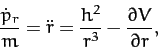(769)

where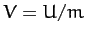. Of course, Equations (768) and (769) are the conventional equations of motion for a particle moving in a central potential--see Chapter 5.Next: Exercises Up: Hamiltonian Dynamics Previous: Constrained Lagrangian Dynamics
Richard Fitzpatrick 2011-03-31